# Potatoes 6

The farmer brought potatoes to the market. In the first hour he sold two-fifths of the potatoes brought, in the second hour he sold five-sixth of the remaining potatoes, and in the third hour he had sold the last 40kg of potatoes. How many kg of potatoes did he sell in the second hour? How many kg did you brought to the market?

Correct result:

b =  200 kg
x =  400 kg

#### Solution:

a = 2/5 x
b = 5/6 •(x-a)
c = 40
x = a+b+c

a = 2/5•x
b = 5/6 •(x-a)
c = 40
x = a+b+c

5a-2x = 0
5a+6b-5x = 0
c = 40
a+b+c-x = 0

a = 160
b = 200
c = 40
x = 400

Our linear equations calculator calculates it.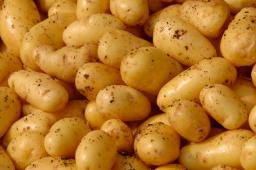We would be pleased if you find an error in the word problem, spelling mistakes, or inaccuracies and send it to us. Thank you!Tips to related online calculators
Need help calculate sum, simplify or multiply fractions? Try our fraction calculator.
Do you have a linear equation or system of equations and looking for its solution? Or do you have quadratic equation?

## Next similar math problems:

• The farmer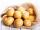The farmer brought potatoes to the market. In the first hour he sold two-fifths of the potatoes brought in, in the second hour he sold five-sixths of the remaining potatoes, and in the third hour, he sold the last 40 kg of potatoes. 1. Express a fraction
• PotatoesFor three days the store sold 1400 kg of potatoes. The first day they sold 100 kilograms of potatoes less than the second day, the third-day three-fifths of what they sold the first day. How many kgs of potatoes sold every day?
• Purchase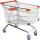Mother bought 5 boxes of milk and 7 kg of potatoes and paid a total CZK 147. Aunt bought 7 boxes of milk and 3 kg of potatoes and paid 131 CZK. What is the price of one carton of milk and 1 kg of potatoes? How CZK together would have saved if bought at th
• Potatoes bags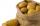I have three bags with 21 kg potatoes. The first bag is 5.5 kg more than in the second bag and the third is 0.5 kg more than in the second bag. Determine how many kgs of potatoes is in each bag.
• Crates 2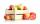In 6 crates is 45 kg of apples In  5 crates is equally In 1 in crate is 3 kg more How many kilograms in each crate?
• Center of gravityThe mass points are distributed in space as follows - specify by coordinates and weight. Find the center of gravity of the mass points system: A1 [1; -20; 3] m1 = 46 kg A2 [-20; 2; 9] m2 = 81 kg A3 [9
• Coffee shop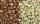To the coffee shop brought 2 types of coffee totally 50 kg. The first type was CZK 220 per kilogram, coffee second type 300 CZK per 1 kg. For all the coffee trader earned CZK 12,000. How many kilograms of coffee of first type and how many kilograms of cof
• The dealer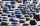The dealer planned to sell 40% of the cars he had in stock in May. However, he sold 20 less cars, ie only 15% of cars. a) How many cars did he have in stock? b) How many cars did he sell in May?
• Apples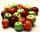Mr. Vesely give to the market his own 48 kg of apples, 40 kg from Mr. Kovar and 72 kg of Mr. Novak. Grossed a total of 1,920 CZK. How must divide income money for apples?
• BucketThe bucket half filled with water weighs 5.55 kg, the full bucket weighs 9.85 kg. How much does the bucket weigh?
• The farmer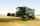The farmer harvested 840 tons of grain in 2006, which was 44% less than in 2005, and one-fifth more than in 2004. How many tons of grain harvested in years 2005 and 2004?
• Barrel 3Barrel with water has a weight 118 kg. When we get off 75% of water it has a weight 35 kg. How many kg has empty barrel?
• SweetsWe want to prepare 5 kg of sweets for 150 CZK. We will mix cheaper candy: 1 kg for 120 CZK and more expensive candy: 1 kg per 240 CZK. How much of this two types of candy is necessary to prepare this mixture?
• Bags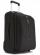The first bag has a weight of 18 kg, the second bag is a one-third lighter than the first and the third bag has three times less the weight of all three bags together. Determine their weights.
• Vegan shopTo the shops brought together 23.2 kg of goods, fruits, vegetables and nuts. fruit was 4.7 kg more than vegetables, nuts was 1.5 kg less than the fruit. Determine the amount of fruits, vegetables and nuts.
• Cooks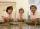Four cooks cleaned 5 kg of potatoes for 10 minutes. How many cook would have to work clean 9 kg of potatoes for 12 minutes?
• Ratio of two unknown numbersTwo numbers are given. Their sum is 30. We calculate one-sixth of a larger number and add to both numbers. So we get new numbers whose ratio is 5:7. Which two numbers were given?